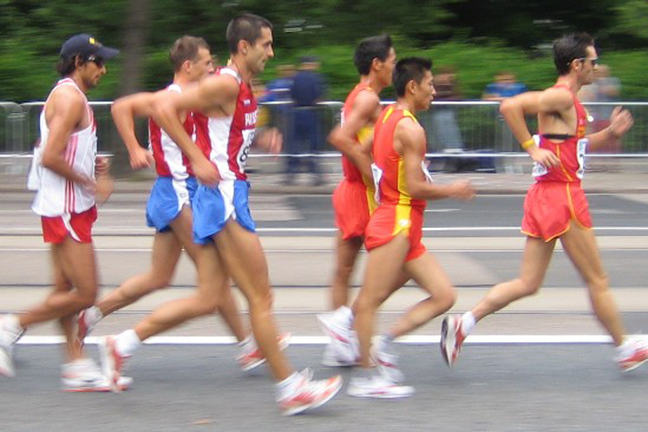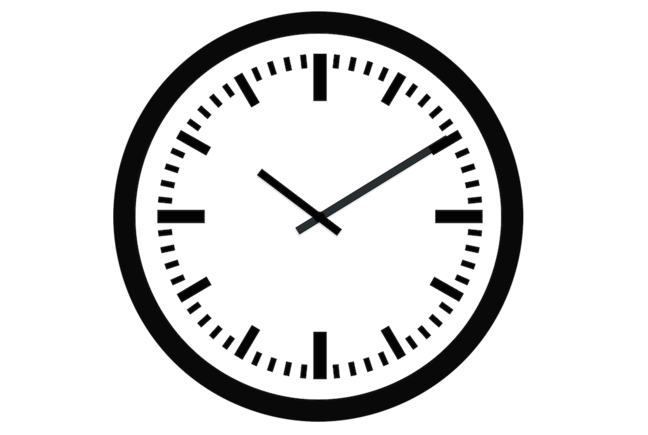## Want to keep learning?

This content is taken from the UNSW Sydney's online course, Maths for Humans: Linear and Quadratic Relations. Join the course to learn more.
1.3

## UNSW SydneyRacewalkers in Helsinki, Finland

# Direct proportionalities from daily life

Direct proportions play a big role in our everyday lives. Here we look at a spectrum of illustrative examples.

In this step you will learn about

• the key definitions for direct proportionality
• direct proportions arising in situations involving speed, money, fuel efficiency of your car, and the role of $$\normalsize{\pi}$$.

## What is a direct proportionality?

If $$\normalsize{x}$$ and $$\normalsize{y}$$ are two variable quantities that satisfy the relation

$\Large{y=kx}$

for some fixed number $$\normalsize{k}$$, then we say that $$\normalsize{y}$$ is directly proportional to $$\normalsize{x}.$$ The number $$\normalsize{k}$$ is called the constant of proportionality. One important way of thinking about this constant $$\normalsize{k}$$ is that it tells us how much $$\normalsize{y}$$ increases by if we increase $$\normalsize{x}$$ by exactly $$\normalsize{1}$$.

We will write

$\Large{y \propto x}$

to express that $$\normalsize{y}$$ is directly proportional to $$\normalsize{x}$$. Note that this symbol however loses an important piece of information: the exact value of the constant of proportionality.

## Distance is proportional to time when walking.

If we walk at a steady pace, say of $$\normalsize{6}$$ km/hour, then we have a basic example of a direct proportionality

$\Large{\operatorname{distance}\propto\operatorname{time}}$

since distance (km) = $$6 \times$$ time (hr).

The constant of proportionality, in this case $$\normalsize{6}$$ km/hr is called the velocity or speed. However an Olympic race-walker will go much faster than this–say twice as fast at $$\normalsize{12}$$ km/hr.

Q1 (E): Describe the relationship between distance and time for an Olympic race walker using this terminology.

Q2 (M): Distance is directly proportional to time. But time is also directly proportional to distance! What is the constant of proportionality in this relationship for Olympic race walkers?

## Time in hours and minutesClock by OpenClipartVectors Pixabay / CC0 Public Domain

Perhaps the most compelling use of direct proportionality is the relationship between hours and minutes:

$\Large{\operatorname{time (min)} = 60 \times \operatorname{time (hr)} .}$

The relation between hours and minutes is so ubiquitous that most people know by rote that 15 minutes is one quarter of an hour. This information is contained within the above equation, which we can see by setting $$\normalsize \mbox{time (hr)} = \frac{1}{4}.$$

${\Large \operatorname{time (min) } = 60 \times \frac{1}{4} = 15 }.$

And how many minutes are in half an hour? The answer is

${\Large \operatorname{time (min)} = 60 \times \frac{1}{2} = 30}.$

Q3 (E): How many minutes are in a day?

## Distance your car can travel vs amount of gas in your tank.© “Only another 1205kms to go” by Tasmin Slater/Flickr CC BY SA 3.0

This is a different kind of proportionality also involving distance–now between how far you can travel in your car and the amount of gas in your tank.

If you have zero gas, you can go nowhere. Let’s say you drive a Holden Commodore – a popular Australian car with a tank capacity of $$\normalsize{73}$$ litres. How far you get depends on whether you are driving in the city or on a freeway, and whether you have the air conditioner on or off etc. But on average, let’s say you can go $$\normalsize{12}$$ km on a litre (L) of gas. Then the relevant proportionality is

${\Large \mbox{distance (km) }\, \propto \,\mbox{gas (L)} }$

with the constant of proportionality the number $$\normalsize{12}$$ km/L, which is a measure of the fuel efficiency of the car.

Q4 (E): Can you drive a Holden Commodore with a full tank of gas from Broken Hill to Dubbo without stopping?

## Diameter vs circumference of a circleThe Earth seen from Apollo 17” Public domain/Wikimedia Commons

Direct proportionalities are also very important in mathematics. For example, it is an important fact that the circumference $$\normalsize{c}$$ of a circle is directly proportional to its diameter $$\normalsize{d}$$. In fact

$\Large{\operatorname{circumference}=\pi \times \operatorname{diameter}}$

where the constant of proportionality is the famous constant $$\normalsize{\pi}$$, roughly $$\normalsize{3.1415926....}$$

So if you double the diameter, you double the circumference. Another implication is that if you increase the Diameter by $$\normalsize{1}$$ unit, then the circumference increases by $$\normalsize{\pi}$$ units. This is independent of the actual size of the circle, which can be surprising.

Q5 (M): If we have a rope tied tight around the equator of the earth, and we want to add some amount or rope so that we can stretch it to be uniformly half a metre off the ground, then how much length to the rope must we add?

A1. One answer would be that $${\normalsize \mbox{distance} \propto \mbox{time}}$$. However this could describe anyone! A more meaningful answer is

$\Large{ \mbox{distance} = 12 \times \mbox{time} }.$

Better still if you include units of distance and time.

A2. We can rearrange $$\normalsize{ \mbox{distance} = 12 \times \mbox{time} }$$ into

${ \Large \mbox{time} = \frac{1}{12} \times \mbox{distance} }.$

So the constant of proportionality is $$\frac{1}{12}$$.

A3. The relationship between days and hours is another example of a direct proportionality:

${\Large \mbox{time (hr)} = 24 \times \mbox{time (days)} .}$

Now we combine the direct proportionality for minutes and hours, with the direct proportionality for hours and days:

${\Large \mbox{time (min)} = 60 \times \mbox{time (hr) } = 60 \times \left(24 \times \mbox{time (days)} \right) }.$

We get a new direct proportionality:

${\Large \mbox{time (min)} = 1440 \times \mbox{time (days)} .}$

So there are $${\normalsize 1440}$$ minutes in a day.

A4. You can expect to travel $${\normalsize 73 \times 12 = 876}$$ km on a single tank of gas. Since you can get from Broken Hill to Dubbo in $${\normalsize 799 - 49 = 750}$$ km, then yes – you can make this trip without stopping.

A5. We are increasing the diameter by $$\normalsize{1}$$, so the circumference must increase by $$\normalsize{\pi}$$, that is, by a bit more than $$\normalsize{3}$$ metres. This is quite unintuitive to many people!